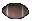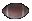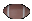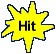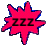Fun Bar Graph Game with Arithmetic Mean   Math Help Tips

- What most people refer to as an 'average value' or central value is called a 'mean value' in Math.

- The arithmetic mean of the three data values {25,25,40} is 30.
The mean = 30 is found by adding up 25+25+40 = 90 and dividing 90 by 3.

- To find the average value of a set of numbers it may help to put the numbers in order.
You can rearrange a data set such as {30,40,20,10} to become {10,20,30,40}.

- The mean of the four data values {10,20,30,40} is 25.
The answer 25 is found by adding up the four values 10+20+30+40 = 100 and dividing 100 by 4.

- In general, the arithmetic mean of a set of data values is equal to
the sum of the data values divided by the number of data values.

- Your Score Report appears after you have made 6 choices.
- Your Game Score is reduced by the number of football hits.

- To slow the game speed repeat tap/click on the word Slider.
- To increase the game speed repeat tap/click on the word Math.
- Speed can also be adjusted with a keyboard's - and + keys.

- Refresh/Reload the web page to restart the game.
- Adjust the sound level of media on your device.

More SliderMath